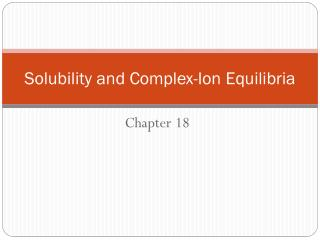Download PresentationSolubility and Complex-Ion Equilibria

# Solubility and Complex-Ion Equilibria

Download Presentation## Solubility and Complex-Ion Equilibria

- - - - - - - - - - - - - - - - - - - - - - - - - - - E N D - - - - - - - - - - - - - - - - - - - - - - - - - - -
##### Presentation Transcript

1. Solubility and Complex-Ion Equilibria Chapter 18

2. To deal quantitatively with an equilibrium, you must know the equilibrium constant. We will look at the equilibria of slightly soluble (or nearly insoluble) ionic compounds and show how you can determine their equilibrium constants. Once you find these values for various ionic compounds, you can use them to answer questions about solubility or precipitation.

3. When an ionic compound is insoluble or slightly soluble, an equilibrium is established: MX(s) M+(aq) + X-(aq) The equilibrium constant for this type of reaction is called the solubility-product constant, Ksp. For the above reaction, Ksp = [M+][X-]

4. Write the solubility-product expression for the following salts: PbCl2 HgCl2

5. The solubility-product constant, Ksp, of a slightly soluble ionic compound is expressed in terms of the molar concentrations of ions in the saturated solution. These ion concentrations are, in turn, related to the molar solubilityof the ionic compound, which is the number of moles of compound that dissolve to give one liter of saturated solution.

6. Exactly 0.133 mg of AgBr will dissolve in 1.00 L of water. What is the value of Ksp for AgBr? Recall: The solubility is given as 0.133 mg/1.00 L, but Ksp uses molarity:

7. Solubility Product Constants

8. An experimenter finds that the solubility of barium fluoride is 1.1 g in 1.00 L of water at 25°C. What is the value of Ksp for barium fluoride, BaF2, at this temperature? Solubility equilibrium: Solubility-product constant expression:

9. When Ksp is known, we can find the molar solubility.

10. Calomel, whose chemical name is mercury(I) chloride, Hg2Cl2, was once used in medicine (as a laxative and diuretic). It has a Ksp equal to 1.3  1018. What is the solubility of Hg2Cl2 in grams per liter? Solubility equilibrium: Solubility-product constant expression:

11. The molar solubility is 6.9 × 10-7M, but we also need the solubility in g/L:

12. We can begin by identifying the value of Ksp for each compound: PbCrO4 1.8 × 10-14 PbSO4 1.7 × 10-8 PbS2.5 × 10-27 Each salt produces two ions, so each has the same expression for the solubility-product constant: Ksp= x2. The solubility will be largest for PbSO4.

13. What effect does the presence of a common ion have on solubility? Given: MX(s) M+(aq) + X-(aq) Qualitatively, we can use Le Châtelier’s principle to predict that the reaction will shift in the reverse direction when M+ or X- is added, reducing the solubility. In the next problem, we will explore this situation quantitatively.

14. What is the molar solubility of silver chloride in 1.0 L of solution that contains 2.0  102 mol of HCl? Given that the Ksp for AgCl at 25°C is 1.8 × 10-10. Next, we construct the ICE chart with the initial [Cl-] = 0.020 M. We then solve for x, the molar solubility.

15. We can use the reaction quotient, Q, to determine whether precipitation will occur. Ksp < QcA precipitate will form One form of kidney stones is calcium phosphate, Ca3(PO4)2, which has a Ksp of 1.0  1026. A sample of urine sample contains 1.0  103M Ca2+ and 1.0  108M PO43 ion. Calculate Qc and predict whether Ca3(PO4)2 will precipitate.

16. Complex-Ion Formation Some cations form soluble complex ions. Their formation increases the solubility of a salt containing those cations. • Metal ions that form complex ions include Ag+, Cd2+, Cu2+, Fe2+, Fe3+, Ni2+, and Zn2+. • Complexing agents, called ligands, are Lewis bases. They include CN-, NH3, S2O32-, and OH-. • In each case, an equilibrium is established, called the complex-ion formation equilibrium.

17. The combination of solubility and complex-ion equilibria can be applied to separate metal ions. Cations can be separated into groups according to their precipitation properties. In each major step, one group of cations precipitates out. After separating the precipitate, the remaining solution is treated with the next reagent, precipitating the next group of cations.